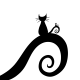# SA6: Parabola Art

This Scripting Algebra (SA) activity uses parabolas to make generative art in p5.js. Learners will investigate existing code to analyze changes in parabolas, and other features of the output.

This activity assumes that the skills from SA1-SA5 were introduced (if-else, logical operators, random(), text(), rect(), variables, loop, pow, for, functions, translate etc.). Counters and resets have been used in previous activities, but are used more with this one.

In this activity, learners will not create their own code at first. They will start with the code below and change one value at a time to analyze its effects. Let learners come up with the approach for analyzing code (give hints as needed). Learners can generate their own code for parabolic art after analysis.

Save work often and have fun (or just start over if you break it).

### Parabola Symmetry

• Analyze the code below by changing one value at a time.
• What happens when numbers are changed? Why?
• Ex: can you make the parabola always face opposite directions?
• Ex: What happens if you change how a,b, and c are created?
• Example Code at p5.js.

See the Pen ParabolaSym by Sophia (@fractalkitty) on CodePen.

Example Concepts: parabolas, variables, coordinate planes, ordered pairs, conditions, loops, random numbers, functions, etc..

## Like what you see?